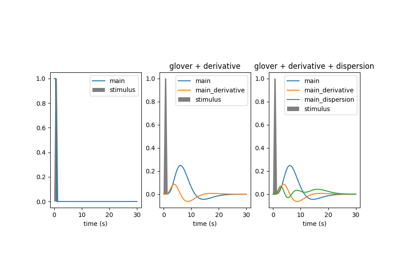Note

This page is a reference documentation. It only explains the function signature, and not how to use it. Please refer to the user guide for the big picture.

3.2.7. nistats.hemodynamic_models.compute_regressor¶

nistats.hemodynamic_models.compute_regressor(exp_condition, hrf_model, frame_times, con_id='cond', oversampling=50, fir_delays=None, min_onset=-24)

This is the main function to convolve regressors with hrf model

Parameters
exp_conditionarray-like of shape (3, n_events)

yields description of events for this condition as a (onsets, durations, amplitudes) triplet

hrf_model{‘spm’, ‘spm + derivative’, ‘spm + derivative + dispersion’,

‘glover’, ‘glover + derivative’, ‘fir’, None} Name of the hrf model to be used

frame_timesarray of shape (n_scans)

the desired sampling times

con_idstring

optional identifier of the condition

oversamplingint, optional

oversampling factor to perform the convolution

fir_delays[int] 1D-array-like, optional

delays (in scans) used in case of a finite impulse response model

min_onsetfloat, optional

minimal onset relative to frame_times (in seconds) events that start before frame_times + min_onset are not considered

Returns
computed_regressors: array of shape(n_scans, n_reg)

computed regressors sampled at frame times

reg_names: list of strings

corresponding regressor names

Notes

The different hemodynamic models can be understood as follows:
• ‘spm’: this is the hrf model used in SPM

• ‘spm + derivative’: SPM model plus its time derivative (2 regressors)

• ‘spm + time + dispersion’: idem, plus dispersion derivative

(3 regressors)

• ‘glover’: this one corresponds to the Glover hrf

• ‘glover + derivative’: the Glover hrf + time derivative (2 regressors)

• ‘glover + derivative + dispersion’: idem + dispersion derivative

(3 regressors)

‘fir’: list or array,

finite impulse response basis, a set of delayed dirac models.

It is expected that spm standard and Glover model would not yield large differences in most cases.

In case of glover and spm models, the derived regressors are orthogonalized wrt the main one.

3.2.7.1. Examples using nistats.hemodynamic_models.compute_regressor¶